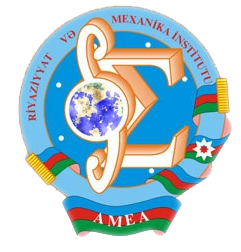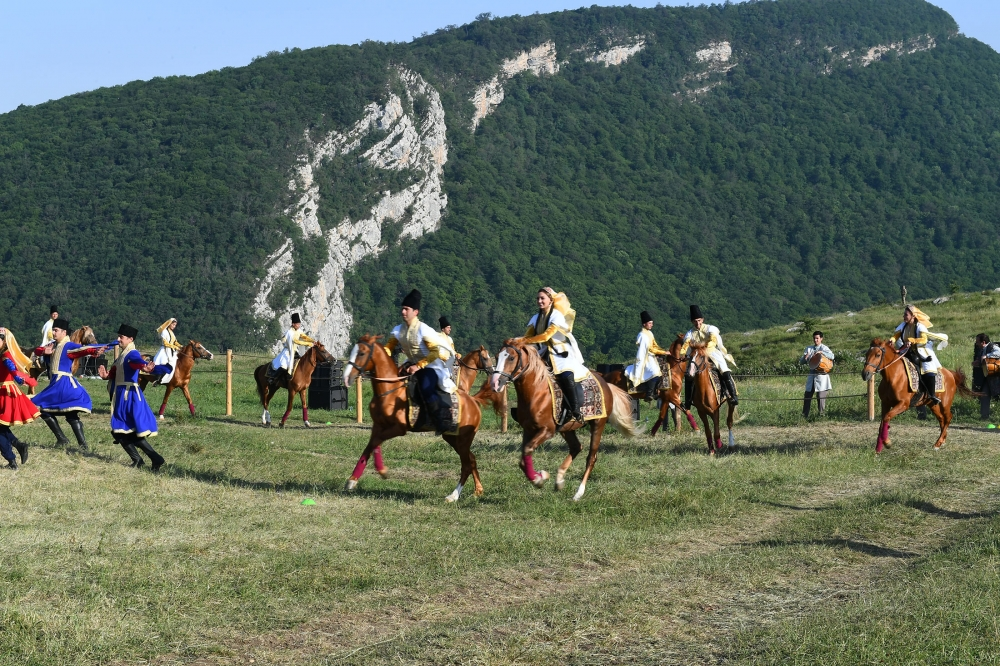Riyaziyyat və Mexanika İnstitutu

## Qarabağ xəbərləri### Şuşada Heydər Əliyev Fondunun təşkilatçılığı ilə “Musiqi irsi və Qarabağ atları Cıdır düzündə” adlı kompozisiya təqdim olunub## Transactions 2004/1/XXIV

 Year: 2004 Volume: 1 Number: XXIV MATHEMATICS Abbasov A.Sh Abstract [PDF] Conditions of correct solvability of a boundary value problem for one class of third order operator-differential equation [PDF] 3 Abdullayev S.K., Agarzayev B.K. Abstract [PDF] Holder weight estimates of singular integrals generated by generalized shift operator [PDF] 9 Akhmedov M.A. Abstract [PDF] The imbedding theorem for a class of weight anisotropic preudonormed spaces[PDF] 19 Aliev A.B., Suleymanov N.A. Abstract [PDF] A mixed problem for some classes quasiiinear equation with Sobolev type[PDF] 27 Aliyev A.R. Abstract [PDF] To the theory of solvability of the second order operator – differential equations with discontinuous coefficients [PDF] 37 Aliyev F.G. Abstract [PDF] Summability of series on root functions in boundary value problems[PDF] 45 Aliyev V.S. Abstract [PDF] On completeness of elementary solutions of the fourth order homogenous operator-differential equations of the elliptic type [PDF] 51 Aliyev S.A., Gasratova M.G. Abstract [PDF] Modeling of Bellman-Harris branching processes[PDF] 59 Aslanov G.I., Rahimov N.Kh. Abstract [PDF] On Green function and distribution of eigenvalues of the second order partial operator- differential equations of elliptic type in half-space [PDF] 67 Aslanova N.M. Abstract [PDF] Integral generalization of the second order matrix differential equation[PDF] 71 Babaev M-B.A. Abstract [PDF] Determination of extremals on the boundary of domain[PDF] 79 Guliyev A.F., Hassanpour A.S. Abstract [PDF] On uniqueness of strong solution of Dirichlet problem for second order quQsilinear elliptic equation [PDF] 85 Gulmamedov V.Ya. Abstract [PDF] On regular solvability of one initial-boundary value problem for third order operator-differential equations [PDF] 93 Huseynov H.M., Latifova A.R. Abstract [PDF] On eigenvalues and eigenfunctions of one class of dirac operators with discontinuous coefficients [PDF] 103 Ilyasov N.A. Abstract [PDF] To the M, Riesz theorem on absolute convergence of the trigonometric Fourier series ldots [PDF] 113 Iskenderov B.A., Mamedova A.J. Abstract [PDF] Mixed problem for Boussinesq equation in the bounded domain and behaviour of its solution as t —t +oo [PDF] 121 Iskenderov N.Sh., Namazov F.A. Abstract [PDF] The scattering problem for hyperbolic system of n equations of the first order on a semi-axis with the n — 1 same velocities [PDF] 137 Jabrailova A.N. Abstract [PDF] On M. V.Keldysh multiple completeness of a system of root vectors of the higher order operator bundle [PDF] 143 Kamali M., Salimov A.A. Abstract [PDF] Horizontal lifts of affinor fields to the tensor bundle[PDF] 149 Khalilov E.H. Abstract [PDF] On approximate solution of one class of boundary integral equations[PDF] 155 Kurbanov V.M., Safarov R.A. Abstract [PDF] On uniform convergence of orthogonal expansions in eigenfunctions of Sturm-Liouville operator [PDF] 161 Mirzoyev R.J. Abstract [PDF] On internal and external hemming indices of k-valued clones II[PDF] 169 Mirzoyev S.S., Jafarov I.J. Abstract [PDF] On solvability of one boundary-value problem for the second order operator-differential equation [PDF] 177 Sadygov M.A. Abstract [PDF] On extremum conditions in problems of mathematical programming[PDF] 187 Yusifov M.R. Abstract [PDF] On correct solvability of a linear nonlocal problem of general form for a class of third order hyperbolic equations [PDF] 197 MECHANICS Agalarov D.H., Mamedov Sh.A., Imamaliyeva D.N. Abstract [PDF] To the problem of wave propagation in elastic medium at movement of cylinder foundation [PDF] 209 Guliyev N.Z. Abstract [PDF] Stability of elastic rod with variable modulus of elasticity situated on non-homogeneous base [PDF] 217 Ismailov R.A. Abstract [PDF] Influence of viscoelastic properties of pipes material on transmission of waves of pressure disturbance at movement of nonequilibrium gases [PDF] 223 Latifov F.S., Seyfullayev F.A. Abstract [PDF] Asymptotic analysis of oscollation eigenfrequency of ortotropic cylindrical shells in infinite elastic medium filled with liquid [PDF] 227 Rassoulova N.B. Abstract [PDF] On one problem of dynamics of rectangular prism[PDF] 231 APPLIED PROBLEMS OF MATHEMATICS AND MECHANICS Gafarov I.A. Abstract [PDF] On one modeling algorithm of random moving of particles along a ring without overtaking [PDF] 237 Ibrahimov V.R. Abstract [PDF] The forward jumping methods[PDF] 251 Melikov T.K. Abstract [PDF] Necessary optimality conditions of quasi-singular controls in Goursat-Darboux systems [PDF] 257
Azərbaycanda COVID-19 ilə bağlı statistika
• Virusa yoluxan

598503

• Sağalan

567385

• Yeni yoluxan

0

• Aktiv xəstə

23114

• Ölüm halı

8004

• Test edilib

5,621,021